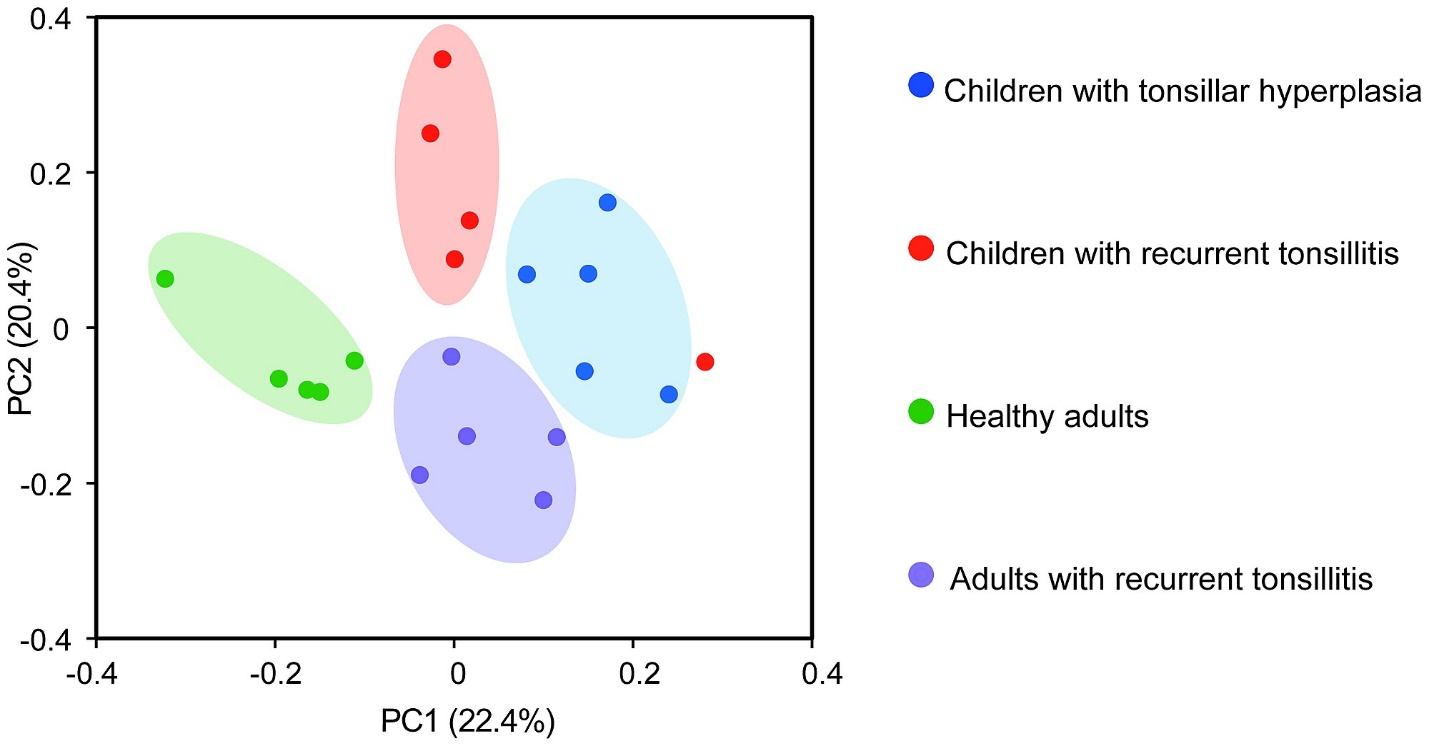/  Top Machine learning interview questions and answers   /  What do you mean by Principal coordinate analysis?## What do you mean by Principal coordinate analysis?

Principal Coordinates Analysis (PCoA,) is a method to explore and to visualize similarities or dissimilarities of data. It starts with a similarity matrix or dissimilarity matrix and assigns for each item a location in a low-dimensional space. PCOA tries to find the main axes through a matrix. It is a kind of eigen analysis and calculates a series of eigenvalues and eigenvectors.

Each eigenvalue has an eigenvector, and there are as many eigenvectors and eigenvalues as there are rows in the initial matrix. By using PCoA we can visualize individual and/or group differences. Individual differences can be used to show outliers.